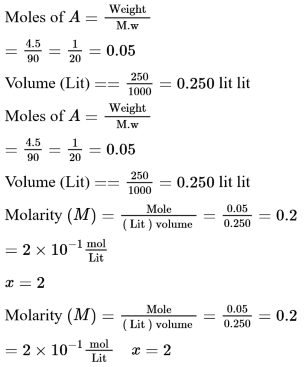# 4.5g of compound A (MW=90) was used to make 250 mL of its aqueous solution.Question:

$4.5 \mathrm{~g}$ of compound $A(\mathrm{MW}=90)$ was used to make $250 \mathrm{~mL}$ of its aqueous solution. The molarity of the solution in $\mathrm{M}$ is $\mathrm{x} \times 10^{-1}$. The value of $\mathrm{x}$ is_____________ . (Rounded off to the nearest integer)

Solution:

(2)